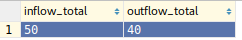# Ecto and sql aliases on fragment

I have a legacy system that has a few queries similar to this one

``````SELECT
sum(CASE WHEN type_id = 1
THEN value END) AS inflow_total,
sum(CASE WHEN type_id = 2
THEN value END) AS outflow_total
FROM transaction
``````

I’m trying to write the same app with elixir but I’m not sure on how to rewrite this using Ecto, so far I have this

``````  def sum_of_inflow_and_outflow(query) do
from t in query, select: fragment("sum(CASE WHEN type_id = 1
THEN value END) AS inflow_total,
sum(CASE WHEN type_id = 2
THEN value END) AS outflow_total")
end
``````

The result should I’m expecting is something like this.When I run this query on the iex it simply returns `50`. Is there a better approach that does not involve sql fragments?

You need to instruct elixir how to decode the result, otherwise ecto has no idea what fields there are. Also, ecto aliases tables, so it’s a good idea to interpolate the fields as ecto understands them. Also, ecto has `sum` as one of the functions, so we can “hoist” it out of the fragment.

``````from t in query, select: %{
inflow_total: sum(fragment("CASE WHEN ? = 1 THEN ? END", t.type_id, t.value)),
outflow_total: sum(fragment("CASE WHEN ? = 2 THEN ? END", t.type_id, t.value))
}``````
3 Likes

Thank you @michalmuskala, thats perfect